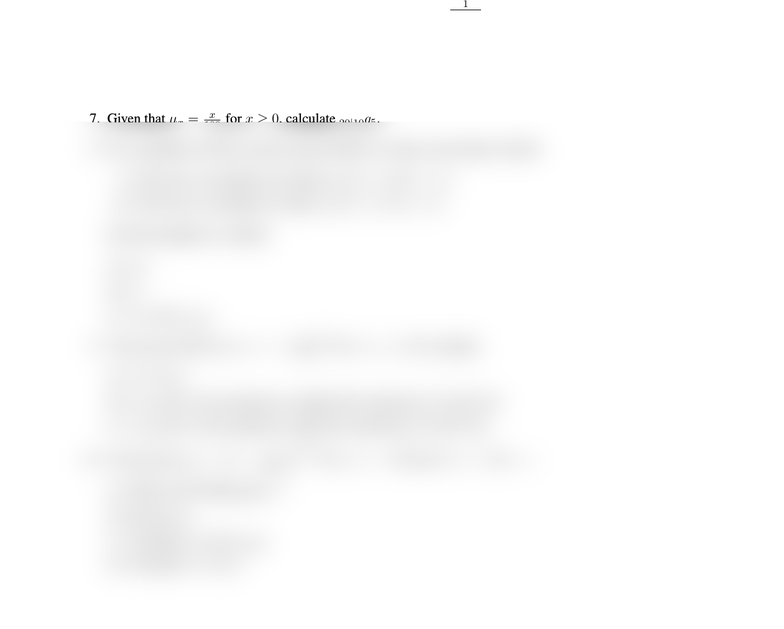# AS 5102 Study Guide - Quiz Guide: Survival Analysis, Problem Set, Life Table

11 views2 pagesTEMPLE UNIVERSITY
AS 3501 ACTUARIAL MODELING I
PROBLEM SET CH.2&3, LM CH.12
Note: The problem set contains optional practice questions from many sources. In particular, it includes
the Exam LTAM/MLC sample questions and textbook exercises.
1. LTAM Sample Qs: 1.1-3.10, LM.1-LM.5 (Note: LM.5 is covered in LM 12.2)
2. LTAM Written Sample Qs: WA.3, WA.24
3. MLC Sample Qs: 13, 21, 22, 28, 32, 59, 65, 66, 98, 106, 116, 120, 131, 136, 145, 155, 161, 168, 171,
188, 189, 200, 201, 207, 219, 223, 267.
4. MLC Written Sample Qs: 1, 3(a).
5. Textbook Exercises: 2.1-2.8, 2.11, 3.2, 3.5, 3.6, LM 12.11-12.16, 12.19-12.25, 12.31-12.37.
6. The force of mortality for a survival model is given by µx=1
110x,0x < 110.
(a) Find out f0(t)and S0(t).
(b) What is the limiting age ωfor this model?
(c) Assess the suitability of this survival model for human mortality.
7. Given that µx=x
100 for x0, calculate 20|10q5.
8. For a population which contains equal numbers of males and females at birth:
(i) The force of mortality for females, µf
x(t) = 0.08,x0
(ii) The force of mortality for males, µm
x(t) = 0.10,x0
For this population, calculate
(a) q50
(b) ˚e0
(c) ˚e20 and ˚e20:10
9. You are given that S0(x) = 1 x
120 2for 0x120, calculate
(a) V ar(T30)
(b) ˚e30:3, the 3-year temporary complete life expectancy of a life (30)
(c) e30:3, the 3-year temporary curtate life expectancy of a life (30)
10. You are given tpx=1t
100x0.5for 0x < 100 and 0t < 100 x.
(a) What is the limiting age ω?
(b) Find out µx
(c) Calculate ˚e30 and ˚e30:20
(d) Calculate V ar(T30)
Unlock document

This preview shows half of the first page of the document.
Unlock all 2 pages and 3 million more documents.